OpenGV A library for solving calibrated central and non-central geometric vision problemsopengv::relative_pose Namespace Reference

The namespace for the relative pose methods. More...

## Classes

class  CentralRelativeAdapter

class  CentralRelativeMultiAdapter

class  CentralRelativeWeightingAdapter

class  MACentralRelative

class  MANoncentralRelative

class  MANoncentralRelativeMulti

class  NoncentralRelativeAdapter

class  NoncentralRelativeMultiAdapter

class  RelativeAdapterBase

class  RelativeMultiAdapterBase

## Functions

translation_t twopt (const RelativeAdapterBase &adapter, bool unrotate, const std::vector< int > &indices)
Compute the translation between two central viewpoints with known relative rotation, and using two correspondences. More...

translation_t twopt (const RelativeAdapterBase &adapter, bool unrotate, size_t index0=0, size_t index1=1)
Compute the translation between two central viewpoints with known relative rotation, and using two correspondences. More...

rotation_t twopt_rotationOnly (const RelativeAdapterBase &adapter, const std::vector< int > &indices)
Compute the rotation between two central viewpoints with pure rotation change, using only two correspondences. More...

rotation_t twopt_rotationOnly (const RelativeAdapterBase &adapter, size_t index0=0, size_t index1=1)
Compute the rotation between two central viewpoints with pure rotation change, using only two correspondences. More...

rotation_t rotationOnly (const RelativeAdapterBase &adapter)
Compute the rotation between two central viewpoints with pure rotation change using Arun's method . Using all available correspondences. More...

rotation_t rotationOnly (const RelativeAdapterBase &adapter, const std::vector< int > &indices)
Compute the rotation between two central viewpoints with pure rotation change using Arun's method . More...

complexEssentials_t fivept_stewenius (const RelativeAdapterBase &adapter)
Compute the complex essential matrices between two central viewpoints using Stewenius' method . Using all available correspondences. More...

complexEssentials_t fivept_stewenius (const RelativeAdapterBase &adapter, const std::vector< int > &indices)
Compute the complex essential matrices between two central viewpoints using Stewenius' method . More...

essentials_t fivept_nister (const RelativeAdapterBase &adapter)
Compute the essential matrices between two central viewpoints using Nister's method . Using all available correspondences. More...

essentials_t fivept_nister (const RelativeAdapterBase &adapter, const std::vector< int > &indices)
Compute the essential matrices between two central viewpoints using Nister's method . More...

rotations_t fivept_kneip (const RelativeAdapterBase &adapter, const std::vector< int > &indices)
Compute the rotation matrices between two central viewpoints using Kneips's method . Only minimal case. More...

essentials_t sevenpt (const RelativeAdapterBase &adapter)
Compute the essential matrices between two central viewpoints using the seven-point algorithm . Using all available correspondences. More...

essentials_t sevenpt (const RelativeAdapterBase &adapter, const std::vector< int > &indices)
Compute the essential matrices between two central viewpoints using the seven-point algorithm . More...

essential_t eightpt (const RelativeAdapterBase &adapter)
Compute the essential matrix between two central viewpoints using the eight-point algorithm [9,10]. Using all available correspondences. More...

essential_t eightpt (const RelativeAdapterBase &adapter, const std::vector< int > &indices)
Compute the essential matrix between two central viewpoints using the eight-point algorithm [9,10]. More...

rotation_t eigensolver (const RelativeAdapterBase &adapter, eigensolverOutput_t &output, bool useWeights=false)
Compute the rotation matrix between two central viewpoints as an iterative eigenproblem . Using all available correspondences. More...

rotation_t eigensolver (const RelativeAdapterBase &adapter, const std::vector< int > &indices, eigensolverOutput_t &output, bool useWeights=false)
Compute the rotation matrix between two central viewpoints as an iterative eigenproblem . More...

rotation_t eigensolver (const RelativeAdapterBase &adapter, bool useWeights=false)
Compute the rotation matrix between two central viewpoints as an iterative eigenproblem . Using all available correspondences. Outputs only the rotation. More...

rotation_t eigensolver (const RelativeAdapterBase &adapter, const std::vector< int > &indices, bool useWeights=false)
Compute the rotation matrix between two central viewpoints as an iterative eigenproblem . Outputs only the rotation. More...

rotations_t sixpt (const RelativeAdapterBase &adapter)
Compute the relative rotation between two non-central viewpoints following Stewenius' method . Assuming exactly 6 correspondences. More...

rotations_t sixpt (const RelativeAdapterBase &adapter, const std::vector< int > &indices)
Compute the relative rotation between two non-central viewpoints following Stewenius' method using 6 correspondences . More...

rotation_t ge (const RelativeAdapterBase &adapter, geOutput_t &output, bool useWeights=false)
Compute the rotation matrix between two non-central viewpoints as an iterative eigenproblem. Using all available correspondences. More...

rotation_t ge (const RelativeAdapterBase &adapter, const std::vector< int > &indices, geOutput_t &output, bool useWeights=false)
Compute the rotation matrix between two non-central viewpoints as an iterative eigenproblem. More...

rotation_t ge (const RelativeAdapterBase &adapter, bool useWeights=false)
Compute the rotation matrix between two non-central viewpoints as an iterative eigenproblem. Using all available correspondences. Outputs only the rotation. More...

rotation_t ge (const RelativeAdapterBase &adapter, const std::vector< int > &indices, bool useWeights=false)
Compute the rotation matrix between two non-central viewpoints as an iterative eigenproblem. Outputs only the rotation. More...

transformation_t seventeenpt (const RelativeAdapterBase &adapter)
Compute the relative pose between two non-central viewpoints following Li's method . Using all available correspondences. More...

transformation_t seventeenpt (const RelativeAdapterBase &adapter, const std::vector< int > &indices)
Compute the relative pose between two non-central viewpoints following Li's method . More...

transformation_t optimize_nonlinear (RelativeAdapterBase &adapter)
Compute the pose between two viewpoints using nonlinear optimization. Using all available correspondences. Works for both central and non-central case. More...

transformation_t optimize_nonlinear (RelativeAdapterBase &adapter, const std::vector< int > &indices)
Compute the pose between two viewpoints using nonlinear optimization. Works for both central and non-central case. More...

## Detailed Description

The namespace for the relative pose methods.

## Function Documentation

 rotation_t opengv::relative_pose::eigensolver ( const RelativeAdapterBase & adapter, eigensolverOutput_t & output, bool useWeights = false )

Compute the rotation matrix between two central viewpoints as an iterative eigenproblem . Using all available correspondences.

Parameters
 [in] adapter Visitor holding bearing-vector correspondences, plus the initial rotation for the optimization. [out] output Returns more complete information (position of viewpoint 2 seen from viewpoint 1, eigenvectors and eigenvalues) [in] useWeights Use weights to weight the summation terms?
Returns
Rotation matrix from viewpoint 2 to viewpoint 1.
 rotation_t opengv::relative_pose::eigensolver ( const RelativeAdapterBase & adapter, const std::vector< int > & indices, eigensolverOutput_t & output, bool useWeights = false )

Compute the rotation matrix between two central viewpoints as an iterative eigenproblem .

Parameters
 [in] adapter Visitor holding bearing-vector correspondences, plus the initial rotation for the optimization. [in] indices Indices of the correspondences used for deriving the rotation matrix. [out] output Returns more complete information (position of viewpoint 2 seen from viewpoint 1, eigenvectors and eigenvalues) [in] useWeights Use weights to weight the summation terms?
Returns
Rotation matrix from viewpoint 2 to viewpoint 1.
 rotation_t opengv::relative_pose::eigensolver ( const RelativeAdapterBase & adapter, bool useWeights = false )

Compute the rotation matrix between two central viewpoints as an iterative eigenproblem . Using all available correspondences. Outputs only the rotation.

Parameters
 [in] adapter Visitor holding bearing-vector correspondences, plus the initial rotation for the optimization. [in] useWeights Use weights to weight the summation terms?
Returns
Rotation matrix from viewpoint 2 to viewpoint 1.
 rotation_t opengv::relative_pose::eigensolver ( const RelativeAdapterBase & adapter, const std::vector< int > & indices, bool useWeights = false )

Compute the rotation matrix between two central viewpoints as an iterative eigenproblem . Outputs only the rotation.

Parameters
 [in] adapter Visitor holding bearing-vector correspondences, plus the initial rotation for the optimization. [in] indices Indices of the correspondences used for deriving the rotation matrix. [in] useWeights Use weights to weight the summation terms?
Returns
Rotation matrix from viewpoint 2 to viewpoint 1.
 essential_t opengv::relative_pose::eightpt ( const RelativeAdapterBase & adapter )

Compute the essential matrix between two central viewpoints using the eight-point algorithm [9,10]. Using all available correspondences.

Parameters
 [in] adapter Visitor holding bearing-vector correspondences.
Returns
Real essential matrix (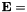skew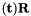, where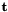is the position of viewpoint 2 seen from viewpoint 1, and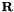is the rotation from viewpoint 2 back to viewpoint 1).
 essential_t opengv::relative_pose::eightpt ( const RelativeAdapterBase & adapter, const std::vector< int > & indices )

Compute the essential matrix between two central viewpoints using the eight-point algorithm [9,10].

Parameters
 [in] adapter Visitor holding bearing-vector correspondences. [in] indices Indices of the correspondences used for deriving the essential matrix.
Returns
Real essential matrix (skew, whereis the position of viewpoint 2 seen from viewpoint 1, andis the rotation from viewpoint 2 back to viewpoint 1).
 rotations_t opengv::relative_pose::fivept_kneip ( const RelativeAdapterBase & adapter, const std::vector< int > & indices )

Compute the rotation matrices between two central viewpoints using Kneips's method . Only minimal case.

Parameters
 [in] adapter Visitor holding bearing-vector correspondences. [in] indices Indices of the correspondences used for deriving the rotation matrices.
Returns
Rotation matrices from viewpoint 2 to viewpoint 1 (maximum 20 solutions).
 essentials_t opengv::relative_pose::fivept_nister ( const RelativeAdapterBase & adapter )

Compute the essential matrices between two central viewpoints using Nister's method . Using all available correspondences.

Parameters
 [in] adapter Visitor holding bearing-vector correspondences.
Returns
Real essential matrices (skew, whereis the position of viewpoint 2 seen from viewpoint 1, andis the rotation from viewpoint 2 back to viewpoint 1, maximum of 10 solutions).
 essentials_t opengv::relative_pose::fivept_nister ( const RelativeAdapterBase & adapter, const std::vector< int > & indices )

Compute the essential matrices between two central viewpoints using Nister's method .

Parameters
 [in] adapter Visitor holding bearing-vector correspondences. [in] indices Indices of the correspondences used for deriving the essential matrices.
Returns
Real essential matrices (skew, whereis the position of viewpoint 2 seen from viewpoint 1, andis the rotation from viewpoint 2 back to viewpoint 1, maximum of 10 solutions).
 complexEssentials_t opengv::relative_pose::fivept_stewenius ( const RelativeAdapterBase & adapter )

Compute the complex essential matrices between two central viewpoints using Stewenius' method . Using all available correspondences.

Parameters
 [in] adapter Visitor holding bearing-vector correspondences.
Returns
Complex essential matrices (skew, whereis the position of viewpoint 2 seen from viewpoint 1, andis the rotation from viewpoint 2 back to viewpoint 1, maximum of 10 solutions).
 complexEssentials_t opengv::relative_pose::fivept_stewenius ( const RelativeAdapterBase & adapter, const std::vector< int > & indices )

Compute the complex essential matrices between two central viewpoints using Stewenius' method .

Parameters
 [in] adapter Visitor holding bearing-vector correspondences. [in] indices Indices of the correspondences used for deriving the essential matrices.
Returns
Complex essential matrices (skew, whereis the position of viewpoint 2 seen from viewpoint 1, andis the rotation from viewpoint 2 back to viewpoint 1, maximum of 10 solutions).
 rotation_t opengv::relative_pose::ge ( const RelativeAdapterBase & adapter, geOutput_t & output, bool useWeights = false )

Compute the rotation matrix between two non-central viewpoints as an iterative eigenproblem. Using all available correspondences.

Parameters
 [in] adapter Visitor holding bearing-vector correspondences, the multi- camera configuration, plus the initial rotation for the optimization. [out] output Returns more complete information (position of viewpoint 2 seen from viewpoint 1, eigenvectors and eigenvalues) [in] useWeights Use weights to weight the summation terms?
Returns
Rotation matrix from viewpoint 2 to viewpoint 1.
 rotation_t opengv::relative_pose::ge ( const RelativeAdapterBase & adapter, const std::vector< int > & indices, geOutput_t & output, bool useWeights = false )

Compute the rotation matrix between two non-central viewpoints as an iterative eigenproblem.

Parameters
 [in] adapter Visitor holding bearing-vector correspondences, the multi- camera configuration, plus the initial rotation for the optimization. [in] indices Indices of the correspondences used for deriving the rotation matrix. [out] output Returns more complete information (position of viewpoint 2 seen from viewpoint 1, eigenvectors and eigenvalues) [in] useWeights Use weights to weight the summation terms?
Returns
Rotation matrix from viewpoint 2 to viewpoint 1.
 rotation_t opengv::relative_pose::ge ( const RelativeAdapterBase & adapter, bool useWeights = false )

Compute the rotation matrix between two non-central viewpoints as an iterative eigenproblem. Using all available correspondences. Outputs only the rotation.

Parameters
 [in] adapter Visitor holding bearing-vector correspondences, the multi- camera configuration, plus the initial rotation for the optimization. [in] useWeights Use weights to weight the summation terms?
Returns
Rotation matrix from viewpoint 2 to viewpoint 1.
 rotation_t opengv::relative_pose::ge ( const RelativeAdapterBase & adapter, const std::vector< int > & indices, bool useWeights = false )

Compute the rotation matrix between two non-central viewpoints as an iterative eigenproblem. Outputs only the rotation.

Parameters
 [in] adapter Visitor holding bearing-vector correspondences, the multi- camera configuration, plus the initial rotation for the optimization. [in] indices Indices of the correspondences used for deriving the rotation matrix. [in] useWeights Use weights to weight the summation terms?
Returns
Rotation matrix from viewpoint 2 to viewpoint 1.
 transformation_t opengv::relative_pose::optimize_nonlinear ( RelativeAdapterBase & adapter )

Compute the pose between two viewpoints using nonlinear optimization. Using all available correspondences. Works for both central and non-central case.

Parameters
 [in] adapter Visitor holding bearing-vector correspondences, the multi-camera configuration, plus the initial values.
Returns
Pose of viewpoint 2 seen from viewpoint 1 (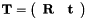, withbeing the position of viewpoint 2 seen from viewpoint 1, andbeing the rotation from viewpoint 2 to viewpoint 1).
 transformation_t opengv::relative_pose::optimize_nonlinear ( RelativeAdapterBase & adapter, const std::vector< int > & indices )

Compute the pose between two viewpoints using nonlinear optimization. Works for both central and non-central case.

Parameters
 [in] adapter Visitor holding bearing-vector correspondences, the multi-camera configuration, plus the initial values. [in] indices Indices of the correspondences used for deriving the pose.
Returns
Pose of viewpoint 2 seen from viewpoint 1 (, withbeing the position of viewpoint 2 seen from viewpoint 1, andbeing the rotation from viewpoint 2 to viewpoint 1).
 rotation_t opengv::relative_pose::rotationOnly ( const RelativeAdapterBase & adapter )

Compute the rotation between two central viewpoints with pure rotation change using Arun's method . Using all available correspondences.

Parameters
 [in] adapter Visitor holding bearing-vector correspondences.
Returns
Rotation from viewpoint 2 to viewpoint 1.
 rotation_t opengv::relative_pose::rotationOnly ( const RelativeAdapterBase & adapter, const std::vector< int > & indices )

Compute the rotation between two central viewpoints with pure rotation change using Arun's method .

Parameters
 [in] adapter Visitor holding bearing-vector correspondences. [in] indices Indices of the correspondences used for deriving the rotation.
Returns
Rotation from viewpoint 2 to viewpoint 1.
 essentials_t opengv::relative_pose::sevenpt ( const RelativeAdapterBase & adapter )

Compute the essential matrices between two central viewpoints using the seven-point algorithm . Using all available correspondences.

Parameters
 [in] adapter Visitor holding bearing-vector correspondences.
Returns
Real essential matrices (skew, whereis the position of viewpoint 2 seen from viewpoint 1, andis the rotation from viewpoint 2 back to viewpoint 1, maximum 3 solutions).
 essentials_t opengv::relative_pose::sevenpt ( const RelativeAdapterBase & adapter, const std::vector< int > & indices )

Compute the essential matrices between two central viewpoints using the seven-point algorithm .

Parameters
 [in] adapter Visitor holding bearing-vector correspondences. [in] indices Indices of the correspondences used for deriving the essential matrices.
Returns
Real essential matrices (skew, whereis the position of viewpoint 2 seen from viewpoint 1, andis the rotation from viewpoint 2 back to viewpoint 1, maximum 3 solutions).
 transformation_t opengv::relative_pose::seventeenpt ( const RelativeAdapterBase & adapter )

Compute the relative pose between two non-central viewpoints following Li's method . Using all available correspondences.

Parameters
 [in] adapter Visitor holding bearing-vector correspondences, plus the multi-camera configuration.
Returns
Pose of viewpoint 2 seen from viewpoint 1 (, withbeing the position of viewpoint 2 seen from viewpoint 1, andbeing the rotation from viewpoint 2 to viewpoint 1).
 transformation_t opengv::relative_pose::seventeenpt ( const RelativeAdapterBase & adapter, const std::vector< int > & indices )

Compute the relative pose between two non-central viewpoints following Li's method .

Parameters
 [in] adapter Visitor holding bearing-vector correspondences, plus the multi-camera configuration. [in] indices Indices of the correspondences used for deriving the rotation matrix.
Returns
Pose of viewpoint 2 seen from viewpoint 1 (, withbeing the position of viewpoint 2 seen from viewpoint 1, andbeing the rotation from viewpoint 2 to viewpoint 1).
 rotations_t opengv::relative_pose::sixpt ( const RelativeAdapterBase & adapter )

Compute the relative rotation between two non-central viewpoints following Stewenius' method . Assuming exactly 6 correspondences.

Parameters
 [in] adapter Visitor holding bearing-vector correspondences, plus the multi-camera configuration.
Returns
Rotations from viewpoint 2 back to viewpoint 1
 rotations_t opengv::relative_pose::sixpt ( const RelativeAdapterBase & adapter, const std::vector< int > & indices )

Compute the relative rotation between two non-central viewpoints following Stewenius' method using 6 correspondences .

Parameters
 [in] adapter Visitor holding bearing-vector correspondences, plus the multi-camera configuration. [in] indices Indices of the six correspondences used for deriving the rotation matrix.
Returns
Rotations from viewpoint 2 back to viewpoint 1
 translation_t opengv::relative_pose::twopt ( const RelativeAdapterBase & adapter, bool unrotate, const std::vector< int > & indices )

Compute the translation between two central viewpoints with known relative rotation, and using two correspondences.

Parameters
 [in] adapter Visitor holding bearing-vector correspondences and known relative rotation. [in] unrotate Set to true if known rotation should be used to unrotate bearing vectors from viewpoint 2 (not required in case of identity rotation). [in] indices Indices of the two correspondences used for deriving the translation.
Returns
Position of viewpoint 2 seen from viewpoint 1.
 translation_t opengv::relative_pose::twopt ( const RelativeAdapterBase & adapter, bool unrotate, size_t index0 = 0, size_t index1 = 1 )

Compute the translation between two central viewpoints with known relative rotation, and using two correspondences.

Parameters
 [in] adapter Visitor holding bearing-vector correspondences and known relative rotation. [in] unrotate Set to true if known rotation should be used to unrotate bearing vectors from viewpoint 2 (not required in case of identity rotation). [in] index0 Index of the first correspondence used for deriving the translation (use default value if only two provided anyway). [in] index1 Index of the second correspondence used for deriving the translation (use default vector if only two provided anyway).
Returns
Position of viewpoint 2 seen from viewpoint 1.
 rotation_t opengv::relative_pose::twopt_rotationOnly ( const RelativeAdapterBase & adapter, const std::vector< int > & indices )

Compute the rotation between two central viewpoints with pure rotation change, using only two correspondences.

Parameters
 [in] adapter Visitor holding bearing-vector correspondences. [in] indices Indices of the two correspondences used for deriving the rotation.
Returns
Rotation from viewpoint 2 to viewpoint 1.
 rotation_t opengv::relative_pose::twopt_rotationOnly ( const RelativeAdapterBase & adapter, size_t index0 = 0, size_t index1 = 1 )

Compute the rotation between two central viewpoints with pure rotation change, using only two correspondences.

Parameters
 [in] adapter Visitor holding bearing-vector correspondences. [in] index0 Index of the first correspondence used for deriving the rotation (use default value if only two provided anyway). [in] index1 Index of the second correspondence used for deriving the rotation (use default value if only two provided anyway).
Returns
Rotation from viewpoint 2 to viewpoint 1.